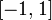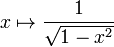# Arc sine function

This article is about a particular function from a subset of the real numbers to the real numbers. Information about the function, including its domain, range, and key data relating to graphing, differentiation, and integration, is presented in the article.
View a complete list of particular functions on this wiki
Template:Angular function convention

## Definition

The arc sine function is denoted$\arcsin$ or$\sin^{-1}$.

For$x$ in the closed interval$[-1,1]$ (i.e.,$-1 \le x \le 1$),$\arcsin x$ is defined as the unique value$y$ in the closed interval$[-\pi/2,\pi/2]$ such that$\sin y = x$.

The arc sine function can be considered the inverse function of the restriction of the sine function to the interval$[-\pi/2,\pi/2]$.

## Key data

Item Value
default domain the closed interval$[-1,1]$
range the closed interval$[-\pi/2,\pi/2]$
first derivative$x \mapsto \frac{1}{\sqrt{1 - x^2}}$, defined on the open interval$(-1,1)$. The left-hand derivative at 1 is not defined (as an infinity, it takes the value$+\infty$). The right-hand derivative at -1 is not defined (as an infinity, it takes the value$+\infty$).